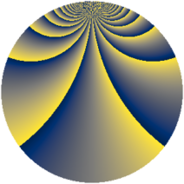# Properties

 Label 2880.2.dwLevel $2880$ Weight $2$ Character orbit 2880.dw Rep. character $\chi_{2880}(181,\cdot)$ Character field $\Q(\zeta_{16})$ Dimension $1280$ Sturm bound $1152$

# Learn more about

## Defining parameters

 Level: $$N$$ $$=$$ $$2880 = 2^{6} \cdot 3^{2} \cdot 5$$ Weight: $$k$$ $$=$$ $$2$$ Character orbit: $$[\chi]$$ $$=$$ 2880.dw (of order $$16$$ and degree $$8$$) Character conductor: $$\operatorname{cond}(\chi)$$ $$=$$ $$64$$ Character field: $$\Q(\zeta_{16})$$ Sturm bound: $$1152$$

## Dimensions

The following table gives the dimensions of various subspaces of $$M_{2}(2880, [\chi])$$.

Total New Old
Modular forms 4672 1280 3392
Cusp forms 4544 1280 3264
Eisenstein series 128 0 128

## Trace form

 $$1280q + O(q^{10})$$ $$1280q - 16q^{22} - 80q^{28} - 80q^{32} - 80q^{34} - 80q^{38} - 16q^{44} - 96q^{52} + 16q^{56} + 144q^{58} - 64q^{59} + 96q^{62} - 96q^{64} - 32q^{67} + 96q^{68} - 64q^{71} + 16q^{74} + 32q^{76} + 32q^{79} + 64q^{80} + 208q^{86} + 160q^{88} + 16q^{94} + 272q^{98} + O(q^{100})$$

## Decomposition of $$S_{2}^{\mathrm{new}}(2880, [\chi])$$ into newform subspaces

The newforms in this space have not yet been added to the LMFDB.

## Decomposition of $$S_{2}^{\mathrm{old}}(2880, [\chi])$$ into lower level spaces

$$S_{2}^{\mathrm{old}}(2880, [\chi]) \cong$$ $$S_{2}^{\mathrm{new}}(64, [\chi])$$$$^{\oplus 6}$$$$\oplus$$$$S_{2}^{\mathrm{new}}(192, [\chi])$$$$^{\oplus 4}$$$$\oplus$$$$S_{2}^{\mathrm{new}}(320, [\chi])$$$$^{\oplus 3}$$$$\oplus$$$$S_{2}^{\mathrm{new}}(576, [\chi])$$$$^{\oplus 2}$$$$\oplus$$$$S_{2}^{\mathrm{new}}(960, [\chi])$$$$^{\oplus 2}$$